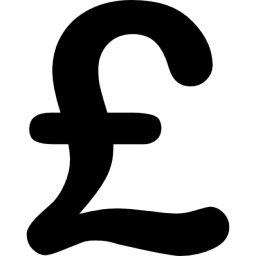# Two-thirds 7531

On a trip to London, Julie spent one-third of the pounds on the first day, two-thirds of the rest on the second day, and 14 pounds on the last day. How many pounds did Julia have on the trip?

p =  63

### Step-by-step explanation:

p/3+2/3·(p-p/3) + 14 = p

0.666667p = 42

p = 42/0.66666667 = 63

p = 63

Our simple equation calculator calculates it.Did you find an error or inaccuracy? Feel free to write us. Thank you!

Tips for related online calculators
Need help calculating sum, simplifying, or multiplying fractions? Try our fraction calculator.
Do you have a linear equation or system of equations and looking for its solution? Or do you have a quadratic equation?